# Section 13.5. Solving Formula Errors

### 13.5. Solving Formula Errors

Errors they occur in the most unexpected places, transforming rows of calculations into unhelpful error codes like # NAME ?, #VALUE! , and #MORON! (OK, that last one doesn't actually appear in Excel, but it might as well, given the sense of defeat and frustration these error codes can give you.) In some cases, you can see how to fix an error just by looking at the formula. However, sometimes the problem isn't so easy to solve, especially if your formulas perform calculations using the results of other formulas. In such cases, you can have a tough time tracking down where the original error occurred.

Excel provides some interesting formula auditing tools a handful of features that you can use to inspect broken formulas or figure out what's going on in really complex ones. These tools make it much easier to fix errors.

With any error, your first step is to identify the error code by using the information listed in Table 8-2 in Section 8.1.5. If the problem isn't immediately obvious, then you can use the Formula Auditing tools to perform the following tasks :

• Evaluate an expression step-by-step, until you hit the error. That way, you know exactly what part of the formula's causing the error.

• Trace the precedents of a formula that's causing an error. Precedents are the cells that a particular formula references. In the formula = A1 + B1 , both A1 and B1 are precedents. If either one of these cells contains an error, then the error gets fed intoand trips upthe formula.

• Trace the dependents of a cell. Dependents are other cells that use the current cell. If one cell has the formula = A1 + B1 , and another cell contains = A1*10 , both these cells are dependents on cell A1. If A1 has an error, it infects both formulas.

• Perform an error check on the entire worksheet. Excel's error check is like a spell check. One by one, it takes you to each cell that has an unresolved problem.

To perform any of these tasks, you'll need the Formulas tab's Formula Auditing section. The following sections explain how you use it to find errors, evaluate formulas piece-by-piece, and trace relationships.

#### 13.5.1. Step-by-Step Evaluation

Complex formulas usually include multiple sub-expressions . Each sub-expression is a piece of any formula that's evaluated separately. It may be an arithmetic operation in parentheses, a nested function, or even just a cell reference. In order to understand what's causing an error in your formula, you need to know which sub-expression caused the problem.

Excel's solution is to provide a featurecalled the Evaluate Formula toolthat evaluates your formula one sub-expression at a time. Using this tool, you can watch as your formula is computed, up until the point where the error occurs.

To watch the step-by-step execution of a formula that contains an error, follow these steps:

1. Move to the cell that contains the formula that's producing the error .

You don't need to highlight the formula, you just need to be in the offending cell.

2. Choose Formulas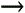Formula AuditingEvaluate Formula .

The Evaluate Formula dialog box appears (Figure 13-18), with the formula in a large, multiline textbox.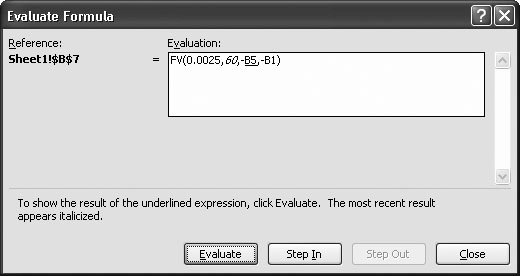Figure 13-18. The first two arguments in this formula have been evaluated. (The second argument  is italicized, indicating that Excel calculated it in the last step.) The next time you click Evaluate, Excel evaluates the third argument, which is underlined . If you want to show the contents of this cell before evaluating it, you can click Step In.

3. Excel underlines the part of the formula that it's about to evaluate. Click the Evaluate button .

Excel evaluates the sub-expression and replaces it with the calculated value. It might replace a cell reference with the cell's actual value, evaluate an arithmetic operator, or execute a function. The value appears in italics, indicating that it's the most recent value that Excel has calculated.

4. Repeat step 3 until the sub-expression that generates the error occurs .

When the error occurs, you'll see the error code appear in your formula. When you click Evaluate again, the error code spreads , encompassing the whole expression or the function that uses it. Consider the ill-fated formula = 1 + 5/0 . The first step (the division) creates a divide-by-zero error, and the formula appears as = 1 + #DIV/0! . But you can't add 1 to an error, so, in the next step, the error spreads to the whole formula, which becomes =#DIV/0! in the end.

5. When the calculation process ends, you can click Restart to repeat the calculation from the beginning .

You can also click Close to stop evaluating the formula and return to your worksheet at any time.

Tip: Step-by-step evaluation isn't just for solving errors. It can also help you understand why a formula doesn't produce the result you expect. You can use the Evaluate Formula dialog box with a formula that doesn't cause an error in exactly the same way as one that does. By watching the calculation proceed step-by-step, you may realize that the order of operations Excel follows is subtly different than the order you expected. You can then edit the formula accordingly .
 GEM IN THE ROUGH Digging Deeper into Linked Formulas Even when you've discovered the sub-expression that's causing the trouble, you still may not have found the root of the problem. If the sub-expression that's causing the error is a reference, it may point to another cell that contains another formula. If it does, then you need to evaluate that formula step-by-step in order to find the real mistake. To evaluate the second formula, you can move to the appropriate cell and start the step-by-step evaluation process by clicking the Evaluate Formula button. However, Excel also provides a useful shortcut that lets you jump from one formula into another. The secret is the Step In and Step Out buttons in the Evaluate Formula dialog box (Figure 13-19). When you're using the Evaluate Formula dialog box, the Step In button becomes available just before you evaluate a sub-expression containing a cell reference. If you click the Step In button at this point, Excel adds a new text box to the dialog box underneath the first one. This new text box shows the contents of the referenced cell. Excel also informs you if the cell contains a formula or a constant (just read the label at the bottom of the Evaluate Formula dialog box). If the cell contains a constant or there's no calculation left to perform, you need to click Step Out to return to the original formula. If the cell does contain a formula, you can click the Evaluate button to start evaluating itone sub-expression at a timeand then click Step Out once you're finished. In fact, Excel lets you dig even deeper into chains of linked formulas. Every time you find a cell reference that points to another formula-holding cell, you can click Step In to show the formula in a new text box. You can continue this process with no practical limit. If you exceed the space available in the Evaluate Formula dialog box, Excel just adds a scroll bar to help you out.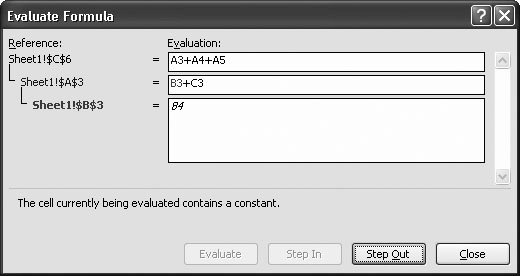Figure 13-19. In this example, the Step In button has taken you three levels deep into a formula. The formula it's evaluating is in the first box; it's A3+A4+A5. However, clicking Step In adds a second box, which reveals that cell A3 itself contains a formula (B3+C3). Finally, another click of Step In shows a third box, which zooms in on the first part of the second formula (B3), and shows that the cell it points to holds the number 84.

#### 13.5.2. Tracing Precedents and Dependents

The Evaluate Formula dialog box is one way you can examine complex formulas' anatomy. However, depending on the complexity of your formulas, you can end up having to move through a long series of steps before you find the problem. In this case, you may be interested in using a different approach, one that uses Excel's ability to graphically trace linked cells. This feature isn't any better or worse than the Evaluate Formula dialog boxit's just another tool that you can use to resolve problems, depending on the situation and your own preference.

First, here's a quick review of how Excel thinks about precedents and dependents. Consider the following formula:

` =A1+B1 `

If this formula is in cell C1, that makes A1 and B1 precedents of C1. In other words, C1 relies on the values in A1 and B1 in order to do its work. If either of these cells contains an error value, the problem spreads into C1. You can say that C1 is the dependent of both A1 and B1.

Excel's tracing features let you see a graphical representation of these relation-shipsin the form of blue arrowsright on your worksheet without needing to look in another window or dialog box.

To see tracing in action, move to a cell that contains one or more cell references, and then choose FormulasFormula AuditingTrace Precedents. Excel displays solid blue arrows that link the cells together. If you click Trace Precedents in the cell C1 that contains that formula = A1 + B1 , you see two arrows. One points from A1 to C1, and the other points from B1 to C1. Figures 13-20 and 13-21 show examples.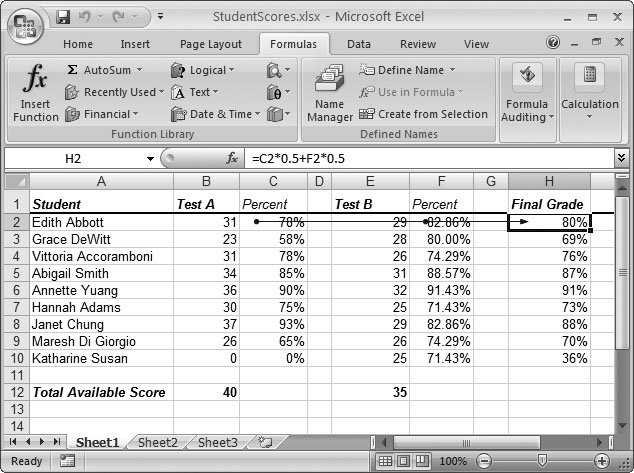Figure 13-20. This example shows the direct precedents of cell H2. As you can see, H2 calculates the student's final grade based on the test results that are stored in cells C2 and F2. Because these two arrows overlap, they appear as one arrow, but you can clearly see two circles, each of which represents the starting point of an arrow (one each on cells C2 and F2).

Note: If a formula references a cell in another worksheet or workbook, Excel draws a dotted line linking your cell to a small grid icon. This icon represents the other worksheet or workbook and can't see the actual cell that the formula links to.Figure 13-21. Excel also lets you trace multiple levels of relationships. Just click the Trace Precedents button again to see whether the precedent cells have other precedents. Here you can see that the test result cells are themselves calculations that rely on other cells. C2 makes its calculations using cells B2 and B12.

The first time you click Trace Precedents, you see the direct precedents . These cells are the ones directly referenced by the current formula. However, these precedents may themselves refer to other cells. To see these cells, click Trace Precedents again.

There's no limit to how many times you can click Trace Precedents. As long as there are more indirect precedents, Excel continues adding arrows. At any point, you can remove a single level of arrows by clicking the Remove Precedent Arrows button, or you can clear everything by choosing FormulasFormula AuditingRemove Arrows.

Tip: Nothing prevents you from tracing the precedents for a bunch of different cells: just move to another cell and repeat the process for each cell you want to trace, one after the other. You can see all the arrows at once, which can make for a tangled worksheet. When you click Remove Arrows, Excel removes all the precedent arrows and any dependent arrows for every cell you've traced. You can remove the arrows for just one cell by moving to it and choosing FormulasFormula AuditingRemove Precedent Arrows.
Formula AuditingTrace Dependents (see Figure 13-22). If you click Trace Dependents and cell A1 is selected, Excel adds an arrow connecting A1 to any other cells that refer to A1.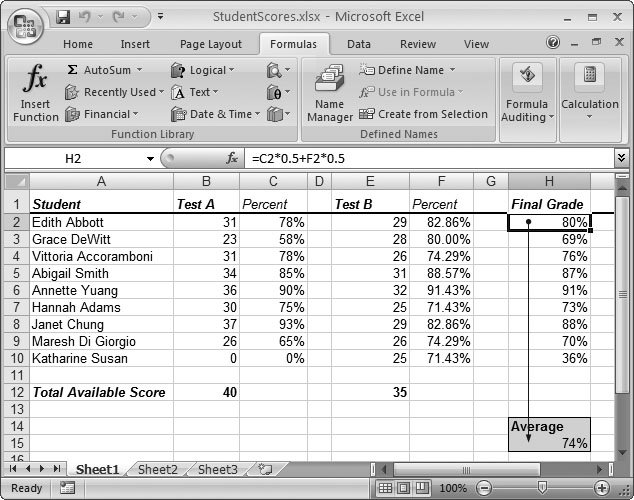Figure 13-22. If you click Trace Dependents on cell H2, Excel indicates that this cell is used in the average calculation in cell H15. However, it isn't the only value that cell H15 uses. To see all the precedents, you'd need to move to H15, and then click Trace Precedents.

Tip: Check out the "Missing CD" page at www.missingmanuals.com to see a screencast (an animated online tutorial) that demonstrates how the precedent and dependent arrows work.

There really isn't a difference between precedent and dependent arrowsthey're just two different ways of looking at the same idea. In fact, every arrow Excel draws connects one precedent to one dependent. Finally, Excel's tracing tools also work with formulas that aren't working (which is important, after all, when it comes to troubleshooting). Figure 13-23 shows how the tool works when your formulas are generating error codes.

#### 13.5.3. Error Checking

Sometimes, you may have a large worksheet that contains a number of errors that are widely distributed. Rather than hunt for these errors by scrolling endlessly, you can jump straight to the offending cells using Excel's error-checking feature.

To perform an error check, follow these steps:

1. Move to the position where you want to start the error check .

If you want to check the entire worksheet from start to finish, click the first cell. Otherwise, go to the location where you want to start checking. As with a spell check, Excel moves from column to column first, and then from row to row. However, the error checker automatically loops back to the beginning of your worksheet, making sure to check every cell before it stops.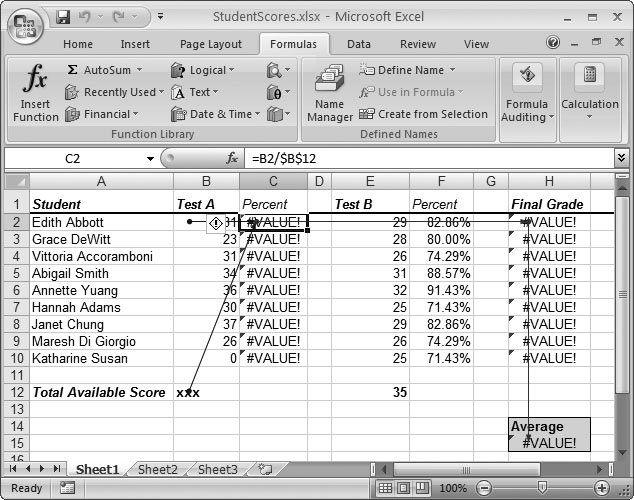Figure 13-23. Excel's tracing features work with any formulaswhether or not they contain an error. But Excel also includes a related feature, Trace Error, which works only with formulas that result in error values. When you select a cell with an error code, and then choose Trace Error, Excel traces all the precedents that lead back to the error by using blue arrows. Then, Excel uses red arrows to indicate how the error spread. In this example, two blue arrows show the precedents of cell C2, where the error occurred. The error then spread to cell H2 and, finally, to the current cell, H15.

2. Choose FormulasFormula AuditingError Checking .

If Excel doesn't find any errors in your worksheet, it displays a message indicating that its work's complete. Otherwise, you see the Error Checking dialog box, as shown in Figure 13-24, which indicates the offending cell and formula. This box also provides a number of options.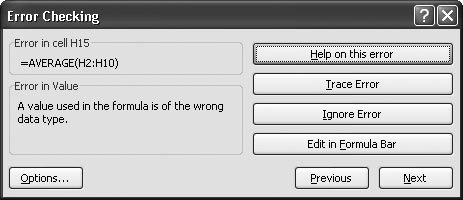Figure 13-24. Excel's error checker helps you scan through a worksheet and quickly jump to the cells that contain errors. You can click the Trace Error button to quickly jump to the Evaluate Formula dialog box and start analyzing the problem.

The Error Checking dialog box contains the following options:

• Next or Previous . Use these buttons to move from one error to the next.

• Help on this Error . Click this button to jump to Excel's online help, which lists common causes of specific errors. It may give you some insight into your own troubles.

• Trace Error . Use this button to open the Evaluate Formula dialog box, where you can move one step at a time through the evaluation of the formula.

• Ignore Error . Click this button to skip the error, and ignore the cell from this point onward. If you want the error checker to pay attention to a cell you've previously decided to ignore, then click the Options button, and then click Reset Ignored Errors.

• Edit in Formula Bar . Use this button to start editing the formula. This choice doesn't close the error checkerinstead, you can click Resume to get back to checking other errors once you've made your change.

• Options . Click this button to open the Excel Options dialog box, with the Formulas section selected. The Formulas section includes a small set of error-checking options under the headings "Error checking" and "Error checking rules" (Section 8.1.5). Ordinarily, you don't need to change any of these error checking options, as the factory settings are stringent enough to ensure that Excel catches all problems.

 GEM IN THE ROUGH Follow the Arrow If you have a complex number-laden spreadsheet with formulas that pull values from all over the place, you may need to scroll around to find the precedents or dependents that interest you. Excel has a trick to help you outjust double-click the appropriate arrow to follow it back to its source cell, no matter where it lies. If you've got a particularly tricky worksheet, it may contain formulas that draw upon values in other worksheets or workbooks. If you double-click the arrow in this situation, then Excel pops up the Go To dialog box (Section 1.3.1), with the information about the source cell already filled in at the top of the list. If you want to follow the arrow to the new worksheet or file, just select the reference (something like, [SuperSecretValues.xlsx]Sheet1!\$A\$3 ), and then click OK.Excel 2007[c] The Missing Manual
ISBN: 596527594
EAN: N/A
Year: 2007
Pages: 173